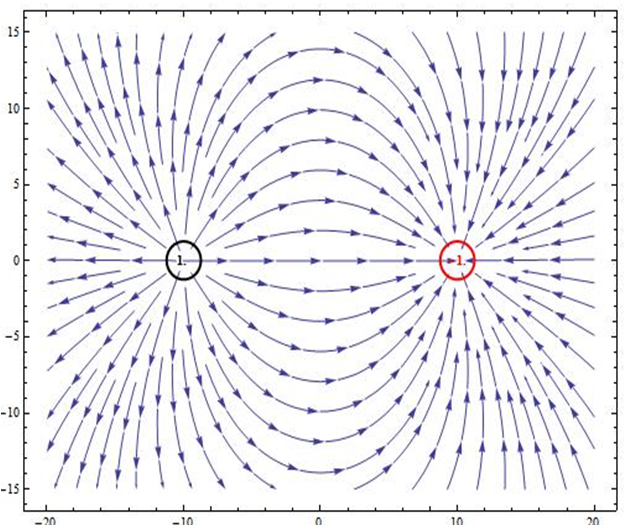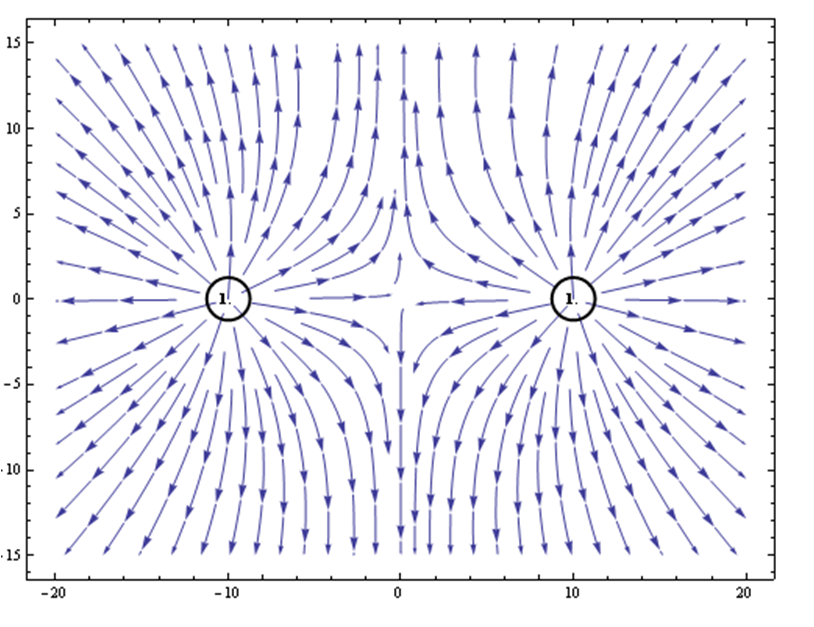## Section29.5Electric Field Lines

When we join electric fields at various points, we get a diagram of directed lines, which are called electric field lines.

The electric field lines of a single positive charge are straight lines in all directions from the charge and the electric field of a single negative charge are straight lines in all directions landing on the charge. See Subsection 29.3.4.

The electric field lines of two or more charges can be constructed from the net electric field of each of the charges. In the exercises in Section 29.4, you have shown that direction of electric field of a two charge system varies in space. Therefore, we construct elctric field lines such that the electric field at a point on the line will be tangent to the line. Figure 29.5.1 shows electric field for two equal charges of opposite types and Figure 29.5.2 shows electric field for two positive charges. What do you think the electric field lines of two negative charges will look like?Figure 29.5.1. Electric field lines for two oppositely charged particles of equal amount. Ref: Wolfram Demonstrations.Figure 29.5.2. Electric field lines for two positive charges of equal amount. Ref: Wolfram Demonstrations.

### Subsection29.5.1Graphical Definition of Electric Field

Using the idea of electric field lines it is possible to introduce a qualitative and more pictorial definition of electric field. According to this approach, we envision space filled with electric field lines from charges according to the following rules.

1. The tangent to an electric field line at a point gives the direction of the electric field at that point.
2. The number of field lines per unit are passing through an imaginary surface normal to the lines corresponds to the magnitude of the electric field.

This graphical definition of electric field is useful in visualizing electric field.